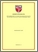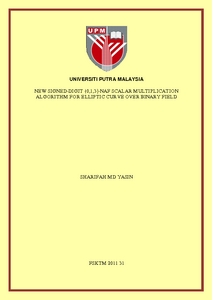# New signed-digit {0,1,3}-NAF scalar multiplication algorithm for elliptic curve over binary field

## Citation

Md Yasin, Sharifah (2011) New signed-digit {0,1,3}-NAF scalar multiplication algorithm for elliptic curve over binary field. PhD thesis, Universiti Putra Malaysia.

## Abstract

Elliptic curve cryptography introduced by Neil Koblitz and Victor Miller in the 80’s. Elliptic curve cryptosystem is very popular recently in comparison with the other public-key cryptosystem such as RSA. This is because elliptic curve cryptosystem uses smaller key size than RSA but it provides equivalent security level with the RSA. Smaller key size means less storage requirement, low power consumption and computing cost. These features are suitable for portable devices such as PDAs, mobile phone, and smartcards. Scalar multiplication is a major operation in elliptic curve cryptosystem. It is the most time consuming and costly operation. It involves with three levels of computations: Scalar arithmetic (Level 1), Point arithmetic (Level 2) and Field arithmetic (Level 3). In the literature, improving scalar representation and efficient point operation can reduce the scalar multiplication cost. In this research, a new left-to-right recoding algorithm is proposed to convert a binary expansion into a new {0,1,3}-NAF representation. The new scalar representation is in base 2 using digit 0, 1 and 3. The special NAF property is adopted in the new {0,1,3}-NAF scalar. Also, a new point tripling formula and algorithm are introduced for elliptic curve over binary field using Lopez-Dahab projective coordinates. The tripling operation is done by computing (2P+P) using one doubling followed by one mixed addition. The tripling cost is (12M+7S) which is cheaper than the cost of computing (2P+P) using traditional method. The new tripling formula saved (2M+2S) when compared with the traditional method. Finally, a new signed-digit {0,1,3}-NAF scalar multiplication algorithm is proposed to utilize the new scalar representation and the new tripling operation. Mathematical analysis is carried out to identify important features, advantages and disadvantages of the new signed-digit {0,1,3}-NAF scalar multiplication algorithm. Cost measurement is based on number of point operations per scalar throughout the execution of the scalar multiplication algorithm. The cost of three scalar multiplication algorithms are compared: double-and-add, additionsubtraction and new signed-digit {0,1,3}-NAF scalar multiplication. By comparison with the double-and-add algorithm, the new signed-digit {0,1,3}-NAF scalar multiplication algorithm has better performance when the value of p is greater than or equal to 2, where p is the number of digit 3 in the new {0,1,3}-NAF representation. By comparison with the addition-subtraction algorithm, the new signed-digit {0,1,3}-NAF scalar multiplication algorithm has better performance when (h2 – h1) > 0 where h1 and h2 are the Hamming weight of the new {0,1,3}-NAF and {-1,0,1}-NAF scalars respectively. Also, from observation, it is effective to use the new signed-digit {0,1,3}-NAF scalar multiplication algorithm when the percentage of the nonzero digit in the binary expansion is less than 75%. In conclusion, performance of a scalar multiplication algorithm depends on the digits used in the scalar representation, and the efficiency of the point operations involved in the scalar multiplication algorithm. Efficiently managed point operations can give optimal number of point operations involved throughout the execution of the scalar multiplication algorithm and can reduced cost of the scalar multiplication.Preview
PDF
FSKTM 2011 31R.pdfView Item